Gaurav Singhal

# Implement Hyperparameter Tuning for Tensorflow2.0

• Jul 31, 2020
• 8,511 Views
• Jul 31, 2020
• 8,511 Views
Data
Data Analytics
Machine Learning
Tensorflow

## Introduction

Remember how you used to tune the radio to improve the channel bandwidth for better sound quality and less background noise?Similarly, in machine learning (ML), you can improve the accuracy of a model (learning algorithm) by tuning hyperparameters, such as the learning rate. Hyperparameters are the parameters whose values are tuned to obtain optimal performance for a model.
Hyperparameter tuning is also known as hyperparameter optimization. Most programmers use exhaustive manual search, which has higher computation cost and is less interactive. TensorFlow 2.0 introduced the TensorBoard HParams dashboard to save time and get better visualization in the notebook.

Model optimization is a continuous process, as shown in the image below: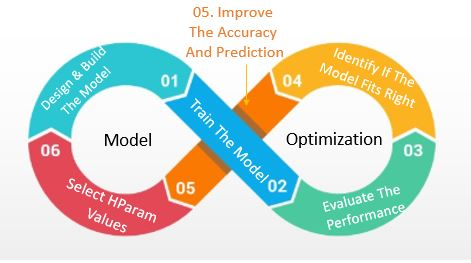This guide will use the inbuilt MNIST dataset, which can easily be loaded from the Keras API database. But before jumping into implementation let's get familiar with some terms.

## What is a Hyperparameter?

In neural network (NN) design, hyperparameter values help the model find weights of a node to understand the pattern of an image, text, or speech more accurately. Their value is set before the training process and doesn't change during the training process.

You can tune values for the following hyperparameters:

1. Number of units and nodes in the dense layer.

2. Learning rate. This controls how quickly the model adapts to the problem. At each iteration, it will determine the step size while moving towards a minimum loss function. Range is between 0.0 and 1.0.

3. Dropout layer. Dropout gives the probability of training a given node in the layer.

4. Optimizer. To reduce loss and get results faster, an optimizer changes the weights and learning rate of a NN. Adam, SDG, rmsprop, and nadam are some of the most commonly used optimizers.

5. L2 regularization. This chooses weights of small magnitude for the model to give a non-spare solution. Regularization is the sum of the square of all feature weights. Lambda is the hyperparameter tuned to strike the balance between simplicity and training-data fit.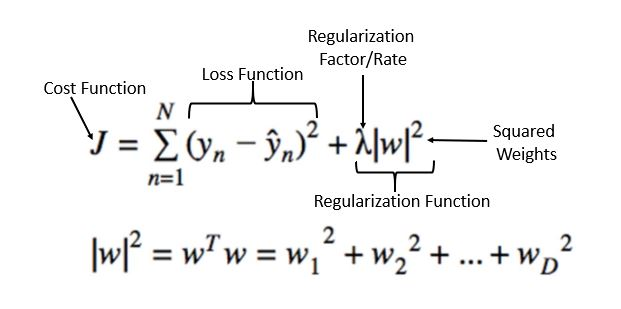Image Source: StackExchange

This can improve your NN performance by reducing overfitting.

1. Epochs. This defines the amount of time that the learning algorithm will take to run through the entire training set. For example, MNIST has 60,000 images, so one epoch means going through all 60,000 images at once.

2. Activation functions. These introduce non-linearity into the output of the neurons. Some examples are given in the image below.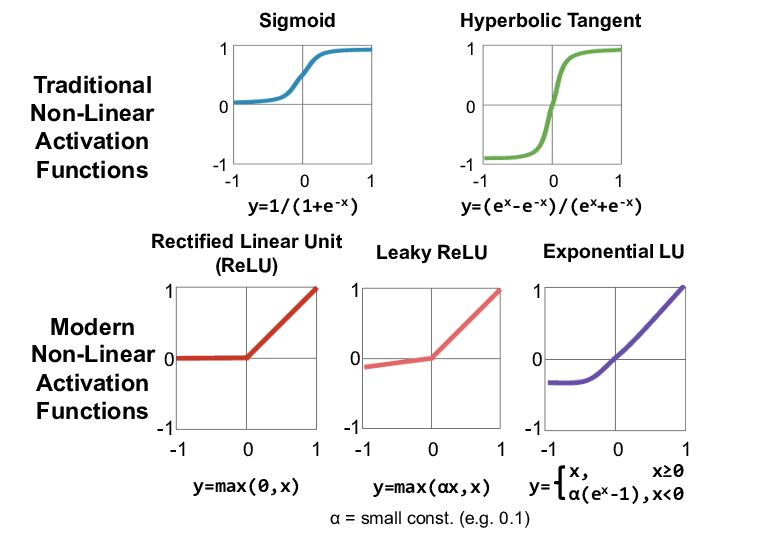Image Source: Arxiv.org

## TensorBoard HParams Dashboard

Often in TensorFlow, while training a model, you just have the screen outputs displaying performance metrics. You can hardly track how the model achieves. To make it easier to understand, optimize, and debug TF programs, TF2.0 has introduced TensorBoard.

TensorBoard helps you visualize TF graphs, plot quantitative metrics, etc. This guide will focus on hyperparameter values using the HParams dashboard. The following steps in the HParams dashboard tools will help you identify the best practices to optimize a set of hyperparameters:

1. Experiment setup and HParams summary
2. Adapt TensorFlow runs to log hyperparameters and metrics
3. Start runs and log them all under one parent directory
4. Visualize the results in TensorBoard's HParams dashboard

## Code Implementation

### Pre-requisites

``1%load_ext tensorboard``
python

Clear any logs from previous runs:

``1!rm -rf ./logs/ ``
python

Import TensorFlow and the TensorBoard HParams plugin:

``````1import tensorflow as tf
2from tensorboard.plugins.hparams import api as hp``````
python

``````1mnist = tf.keras.datasets.mnist
2
4x_train, x_test = x_train / 255.0, x_test / 255.0``````
python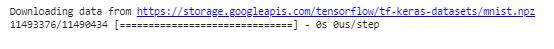### 1. Experiment Setup and HParams Experiment Summary

Experiment with four hyperparameters: in the model:

1. Number of units in the first dense layer
2. Dropout rate in dropout layer
3. Optimizer
4. L2 Regularizer
``````1HP_NUM_UNITS = hp.HParam('num_units', hp.Discrete([256, 512]))
2HP_DROPOUT = hp.HParam('dropout', hp.RealInterval(0.5, 0.6)
4HP_L2 = hp.HParam('l2 regularizer', hp.RealInterval(.001,.01))
5
6METRIC_ACCURACY = 'accuracy'
7
8with tf.summary.create_file_writer('logs/hparam_tuning').as_default():
9  hp.hparams_config(
10    hparams=[HP_NUM_UNITS, HP_DROPOUT, HP_OPTIMIZER,HP_L2],
11    metrics=[hp.Metric(METRIC_ACCURACY, display_name='Accuracy')],
12  )``````
python

### 2. Adapt TensorFlow Runs to Log Hyperparameters and Metrics

The model contains two dense layers with a dropout layer between them. The hyperparameters are not hardcoded, although the training code will be similar. All the hyperparameters are provided in the `hparams` dictionary.

``````1def train_test_model(hparams):
2  model = tf.keras.models.Sequential([
3    tf.keras.layers.Flatten(),
4    tf.keras.layers.Dense(hparams[HP_NUM_UNITS], kernel_regularizer=tf.keras.regularizers.l2(0.001), activation=tf.nn.relu),
5    tf.keras.layers.Dropout(hparams[HP_DROPOUT]),
6    tf.keras.layers.Dense(10, activation=tf.nn.softmax),
7  ])
8  model.compile(
9      optimizer=hparams[HP_OPTIMIZER],
10      loss='sparse_categorical_crossentropy',
11      metrics=['accuracy'],
12  )
13
14  model.fit(x_train, y_train, epochs=2)
15  _, accuracy = model.evaluate(x_test, y_test)
16  return accuracy``````
python

For each run, log an HParams summary with the hyperparameters and final accuracy:

``````1def run(run_dir, hparams):
2  with tf.summary.create_file_writer(run_dir).as_default():
3    hp.hparams(hparams)  # record the values used in this trial
4    accuracy = train_test_model(hparams)
5    tf.summary.scalar(METRIC_ACCURACY, accuracy, step=2)``````
python

### 3. Start Runs and Log them All Under One Parent Directory

You can now try multiple experiments, training each one with a different set of hyperparameters.

``````1session_num = 0
2
3for num_units in HP_NUM_UNITS.domain.values:
4  for dropout_rate in (HP_DROPOUT.domain.min_value, HP_DROPOUT.domain.max_value):
5    for l2 in (HP_L2.domain.min_value, HP_L2.domain.max_value):
6      for optimizer in HP_OPTIMIZER.domain.values:
7        hparams = {
8            HP_NUM_UNITS: num_units,
9            HP_DROPOUT: dropout_rate,
10            HP_L2: l2,
11            HP_OPTIMIZER: optimizer,
12        }
13        run_name = "run-%d" % session_num
14        print('--- Starting trial: %s' % run_name)
15        print({h.name: hparams[h] for h in hparams})
16        run('logs/hparam_tuning/' + run_name, hparams)
17        session_num += 1``````
python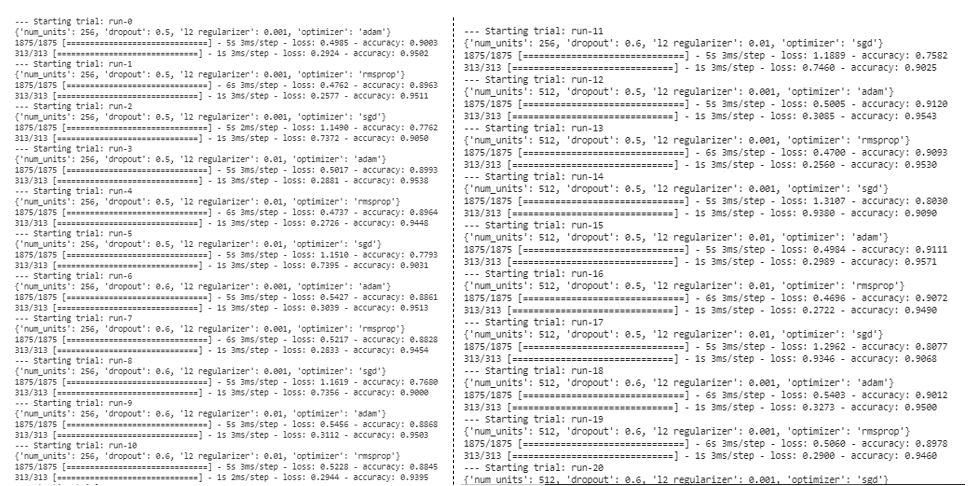### 4. Visualize the Results in TensorBoard's HParams Dashboard

Open the HParams Dashboard. Once TensorBoard starts, click HParams at the top.

``1%tensorboard --logdir logs/hparam_tuning``
python

#### Table View

Table View lists the name of the session and performance metrics of the hyperparameters. The square checkboxes allow you to limit the view of the metrics.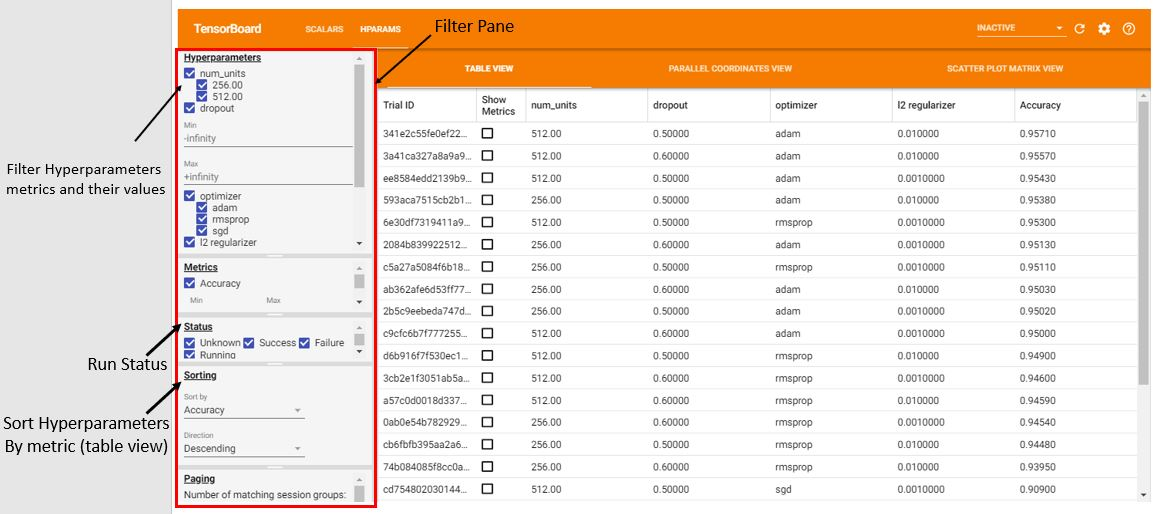#### Parallel Coordinate View

This view displays a run as a line (color-coded) that passes through the axis of each hyperparameter, and metrics at the end show the accuracy. It is important to know which set of hyperparameters is more important. If you place the mouse pointer on any axis, the run that passes through will get highlighted. You can reorder the axes by dragging them.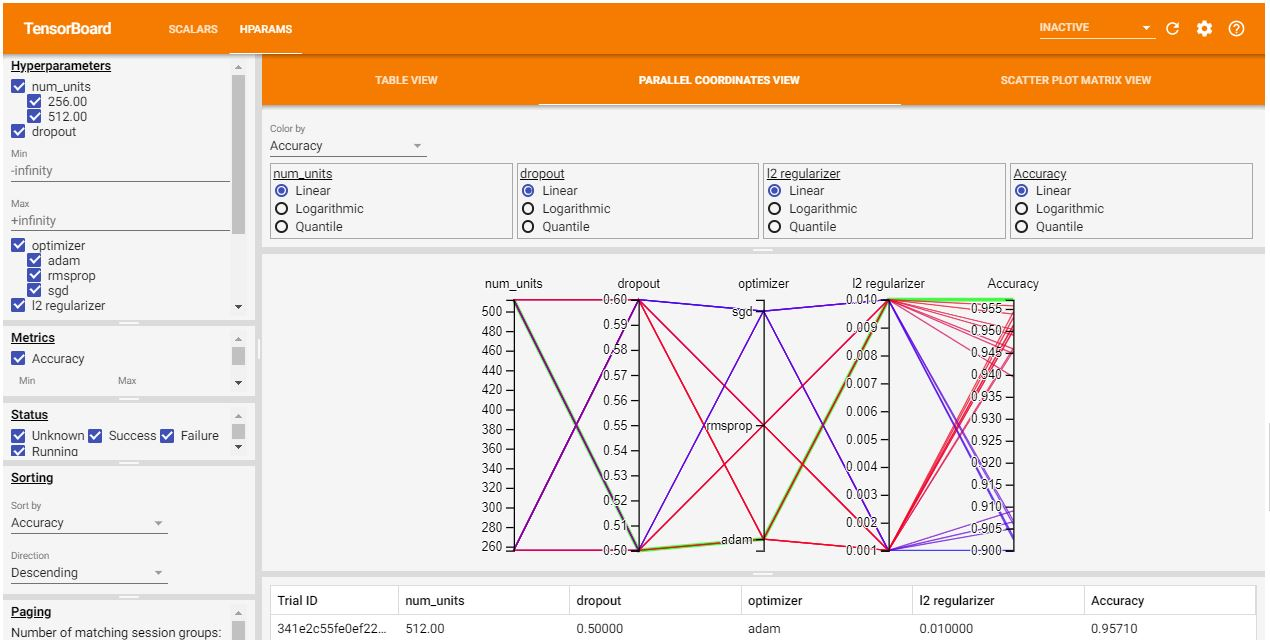#### Scatter Plot View

This view is used to identify the correlation between each metric. Click or hover over a session group to highlight the session across plots.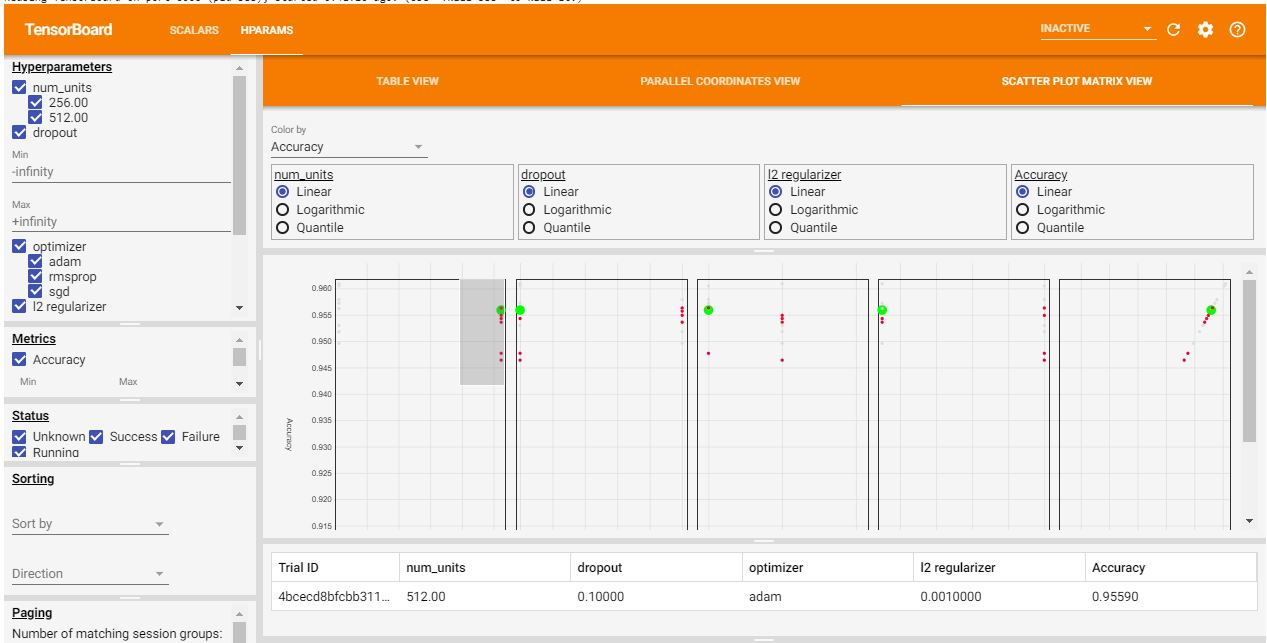## Conclusion

Sorting the accuracy in descending order shows that the most optimized model has 512 units with a dropout rate of 0.5 and Adam optimizer with an L2 regularization rate of 0.01 and accuracy of 95.710%. The model can be optimized further. You can include more performance metrics for better visualization and understanding.

This guide gave a brief introduction to TensorBoard. TensorBoard's HParams dashboard provides amazing visualization to help you understand which hyperparameter can be further fine-tuned to make your NN model more accurate and reliable.

You can explore other TensorBoard features like graphs, projector, etc., here.

I hope you enjoyed learning. If you have any queries, feel free to contact me at CodeAlphabet.